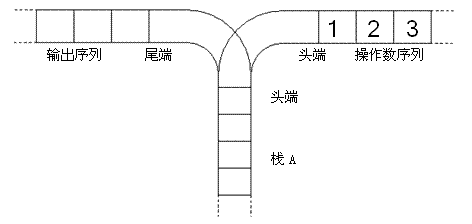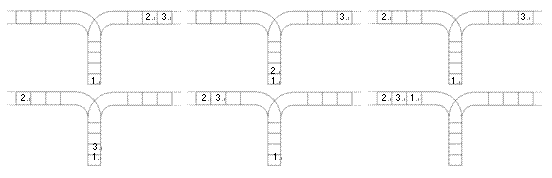# 2021.09.24

m 个整数表示答案，用换行隔开。

## 样例

输入：
10 3
1 9 2 60 8 17 11 4 5 14
1 5 9

1
8
5

## 思路和知识点

vector基本用法
1. vector <int> array (N, i); 新建一个初始长度为N，N个数据为i的vector数组
2. array.push_back(a); 将a元素添加到数组array的末尾，并增加数组长度
3. array.size(); 返回数组的长度
4. array.resize(n, m); 重新将数组长度设置为n，若原数组比n长，则删除多余元素，若短，新增加的数组初始化为m
5. array.begin(); 返回array数组的首元素，也就是array的指针
6. array.end(); 返回array数组末尾下一个元素的指针

## AC代码

#include <iostream>
#include <vector>
using namespace std;
int main()
{
int n, m;
vector <int> student; // 创建vector数组
int temp = 0;
cin>>n>>m;
for(int i = 0; i < n; i++)
{
cin>>temp;
student.push_back(temp); // 将temp压入动态vector数组的末尾，并且vector数组的长度+1
}
for(int i = 0; i < m; i++)
{
cin>>temp;
cout<<student[temp - 1]<<"\n";// 数组一般是从0开始存储数据，而我们询问学号是从1开始寻找
}
return 0;
}

## 题目描述

• 1 i j k：在第 $i$ 个柜子的第 $j$ 个格子存入物品 $k(0\le k\le 10^9)$。当 $k=0$ 时说明清空该格子。
• 2 i j：查询第 $i$ 个柜子的第 $j$ 个格子中的物品是什么，保证查询的柜子有存过东西。

## 样例

输入：
5 4
1 3 10000 114514
1 1 1 1
2 3 10000
2 1 1

114514
1

vector相关操作

## AC代码

#include <iostream>
#include <cstdio>
#include <vector>
using namespace std;
int main()
{
int n, q, op;
int i, j, k;
cin>>n>>q;
vector < vector <int> > block (n + 1);// 此时仅仅是开了一个长度为n的一维数组
for(int count = 0; count < q; count++)
{
cin>>op;
if(op == 1)
{
cin>>i>>j>>k;
if(block[i].size() < j + 1)// 如果柜子里格子不足，那么就扩展第i个柜子的格子到j + 1
{
block[i].resize(j + 1);
}
block[i][j] = k;
}
else
{
cin>>i>>j;
cout<<block[i][j]<<"\n";
}
}
return 0;
}

## 思路和知识点

C语言提供了栈的相关快捷操作<stack>
1. stack <int> s; 新建一个栈
2. s.push(a); 将元素a压入栈
3. s.pop(); 将s的栈顶元素弹出
4. s.top(); 查询s的栈顶元素
5. s.size(); 查询s的元素个数
6. s.empty(); 查询s是否为空

## 手写栈模板(请根据实际情况进行修改)

int stack;
int p = 0;// 指针，指向的是下一个栈元素
void push(int x)// 压栈操作
{
stack[p++] = x;
}
void pop()// 弹出栈顶元素
{
stack[--p] = 0;
}
int top()// 查询栈顶元素
{
return stack[p - 1];
}

## 题目描述

1. 空串合法；
2. 如果A和B合法，那么AB合法；
3. 如果A合法(A)和[A]都合法

## 样例

输入:
3
([])
(([()])))
([()[]()])()

Yes
No
Yes

## AC代码

#include <iostream>
#include <string>
#include <cstdio>
using namespace std;
int stack;
int p = 0;// 栈顶指针,指的是下一个待插入的数组位置
void push(int x)// 压栈操作
{
stack[p++] = x;
}
void pop()
{
stack[--p] = 0;
}
int top()
{
return stack[p - 1];

}
char judge(char a)// 因为要让右边的括号匹配左边的括号，所以说对于每一种右括号都应该返回相对应的左括号形式
{
if(a == ')')
{
return '(';
}
if(a == ']')
{
return '[';
}
if(a == '}')
{
return '{';
}
return '/0';
}
int main()
{
int num;
cin>>num;
string str;
getline(cin, str);// C++在使用cin输入时，光标停留在数字行的末尾，如果此时用getline读取一行，那么将读取到空行
while(num--)
{
while(p != 0)
{
pop();
}
getline(cin, str);// 这里才是真正的去读入字符串
for(int i = 0; i < str.size(); i++)
{
if(p == 0)
{
push(str[i]);// 栈空则压入数据
continue;
}
if(judge(str[i]) == top())// 栈顶左括号与右括号匹配
{
pop();
}
else
{
push(str[i]);
}
}
if(p == 0)
{
cout<<"Yes\n";
}
else
{
cout<<"No\n";
}
}
return 0;
}

# 2021.09.26

## 样例

输入：
3.5.2.-*7.+@

16  

## AC代码

#include <iostream>
#include <cstdio>
using namespace std;
int stack;
int p = 0;
void push(int x)
{
stack[p++] = x;
}
void pop()
{
stack[--p] = 0;
}
int top()
{
return stack[p - 1];
}
int main()
{
int x, y; // x, y是用于存放两个算数的临时变量
int sum = 0;
char ch;
do
{
ch = getchar();
if(ch <= '9' && ch >= '0')
{
sum = sum * 10 + (ch - '0');
}
else if(ch == '.')
{
push(sum);
sum = 0;
}
else if(ch != '@')
{
x = top();
pop();
y = top();
pop();
switch (ch)
{
case '+':
push(x + y);
break;
case '-':
push(y - x);
break;
case '*':
push(x * y);
break;
case '/':
push(y / x);
break;
//default:
//break;
}
}
} while (ch != '@');
printf("%d", top());
return 0;
}

## 样例

输入：
2*(x+y)/(1-x)@

YES

(25+x)*(a*(a+b+b)@

NO

## AC代码

#include <iostream>
#include <string>
#include <cstdio>
using namespace std;
int stack;
int p = 0;
void push(int x)
{
stack[p++] = x;
}
void pop()
{
stack[--p] = 0;
}
int top()
{
return stack[p - 1];
}
int main()
{
string s;
getline(cin, s);
for(int i = 0; i < s.size(); i++)
{
if(s[i] == '(')
{
push(s[i]);
}
else if(s[i] == ')')
{
if(top() == '(')
{
pop();
}
else
{
push(s[i]);
}
}
}
if(p != 0)
{
printf("NO");
}
else
{
printf("YES");
}
return 0;
}

## 7. P1044 (NOIP2003 普及组)栈 - 洛谷 - 普及

### 题目描述1. 将一个数，从操作数序列的头端移到栈的头端（对应数据结构栈的 push 操作）
2. 将一个数，从栈的头端移到输出序列的尾端（对应数据结构栈的 pop 操作）（原始状态如上图所示）

## 样例

输入：
3

5

## AC代码

#include <iostream>
#include <cstdio>
using namespace std;
int k;
int main()
{
int n;
cin>>n;
k = 1; k = 1;
for(int i = 2; i <= n; i++)
{
for(int j = 0; j < i; j++)
{
k[i] += k[j] * k[i - j - 1];
}
}
cout<<k[n];
return 0;
}

## 题目描述

$n$ 个人围成一圈，从第一个人开始报数,数到 $m$ 的人出列，再由下一个人重新从 $1$ 开始报数，数到 $m$ 的人再出圈，依次类推，直到所有的人都出圈，请输出依次出圈人的编号。

## 样例

输入：
10 3

3 6 9 2 7 1 8 5 10 4

## AC代码

#include <iostream>
#include <cstdio>
using namespace std;
int queue;
int head = 0, tail = 0;
void push(int x)
{
queue[tail++] = x;
}
void pop()
{
}
int front()
{
}
int main()
{
int n, m;
cin>>n>>m;
if(n == 0 && m == 0)
{
cout<<"1";
return 0;
}
for(int i = 1; i <= n; i++)
{
push(i);
}
{
for(int i = 1; i < m; i++)
{
push(front());
pop();
}
cout<<front()<<" ";
pop();
}
return 0;
}